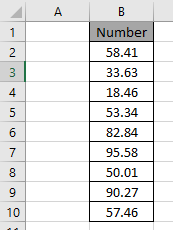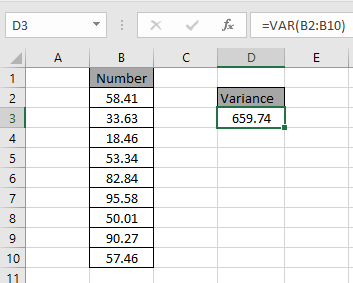# How to use the VAR Function in Excel

In this article, we will learn about how to use VAR function in excel to get the variance of the data.

In simple words, get the estimate variation of sample of the data. Use VARP or newer VAR.P function if data represents the entire population.

VAR function in Excel used to find the VARIANCE of the data set in Excel.

Syntax:

=VAR (number1, [number2],...)

Texts and logical values are ignored by VAR function.

Example:

Let’s use it in an example to understand it.Here we have an array of numbers need to find the variance

Use the formula to get the variance for the range

=VAR(B2:B10)Variance for the following data is 659.74.
Variance Table

 Name Data set Text and logicals VAR Sample Ignored VARP Population Ignored VAR.S Sample Ignored VAR.P Population Ignored VARA Sample Evaluated VARPA Population Evaluated

Excel VAR function is not used popularly. Excel replaced the use of VAR function with VAR.S is used to get the variance of the data.

Hope you understood how to use VAR function in Excel. Explore more articles on Excel Statistical functions here. Please feel free to state your queries or feedback in the comment box below.

Popular Articles:

50 Excel Shortcuts to Increase Your Productivity

How to use the VLOOKUP Function in Excel

How to use the COUNTIF function in Excel 2016

How to Use SUMIF Function in Excel

Terms and Conditions of use

The applications/code on this site are distributed as is and without warranties or liability. In no event shall the owner of the copyrights, or the authors of the applications/code be liable for any loss of profit, any problems or any damage resulting from the use or evaluation of the applications/code.